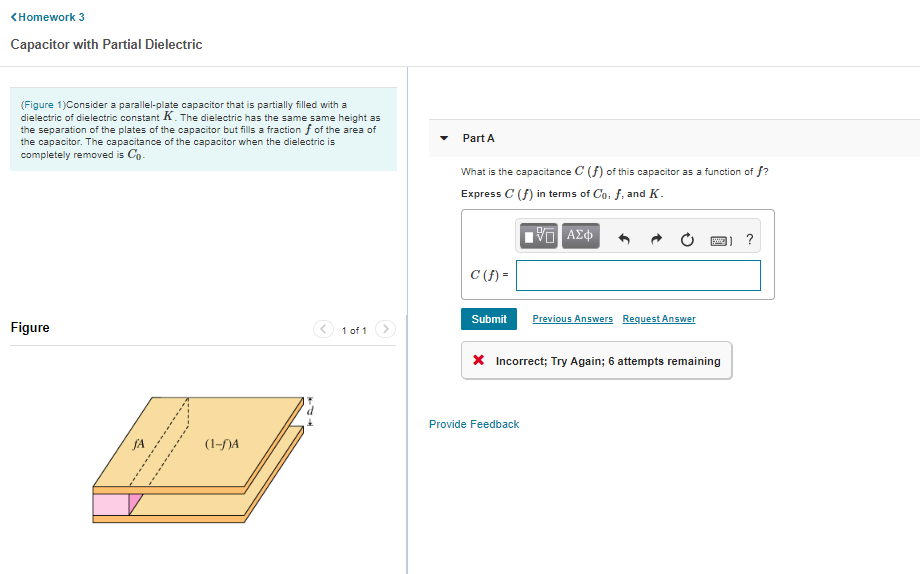# (Solved): ...Need physics help

<Homework 3 Capacitor with Partial Dielectric (Figure 1) Consider a parallel-plate capacitor that is partially filled with a dielectric of dielectric constant K. The dielectric has the same same height as the separation of the plates of the capacitor but fills a fraction f of the area of the capacitor. The capacitance of the capacitor when the dielectric is completely removed is Co- Part A What is the capacitance C (f) of this capacitor as a function of f? Express C (f) in terms of Cof, and K. % AD mo ? C(F)- Submit Previous Answers Request Answer Figure 1 of 1 X Incorrect; Try Again; 6 attempts remaining Provide Feedback (1-9A

We have an Answer from Expert